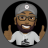# AddChartBubble when condition is met#### Branch

##### Member
VIP
I would like to add a Chart Bubble to the code below; when the opentime is true place a Chart bubble at the high of the 5 minute close. For this time frame I would like the bubble text to show the word "Now" (I can change the text later.)

Code:
``````input opentime = 0400;
input ORend = 0405;
def na = Double.NaN;
#
# Check if the opening range time is now
#
def ORActive = if GetLastDay() == GetDay() and SecondsFromTime(opentime) >= 0 and SecondsFromTime(ORend) < 0 then 1 else 0;
#
# Track the OR high and low
#
def ORHigh = if ORActive then high else na;
def ORLow = if ORActive then low else na;
def ORLow2 = if ORActive then low else na;
#
# Plot the OR high and low
#
plot OHIGH = if GetLastDay() != GetDay() or !ORActive then na else HighestAll(ORHigh);
plot OLOW = if GetLastDay() != GetDay() or !ORActive then na else LowestAll(ORLow);
plot OLOW2 = if GetLastDay() != GetDay() or !ORActive then na else LowestAll(ORLow);
plot HIGH = if GetLastDay() != GetDay() or SecondsFromTime(ORend) < 0 then na else HighestAll(ORHigh);
plot LOW = if GetLastDay() != GetDay() or SecondsFromTime(ORend) < 0 then na else LowestAll(ORLow);
#
# Formatting
#
OHIGH.SetStyle(Curve.SHORT_DASH);
OLOW.SetStyle(Curve.SHORT_DASH);
OLOW2.SetStyle(Curve.SHORT_DASH);
OHIGH.SetDefaultColor(Color.GREEN);
OLOW.SetDefaultColor(Color.RED);
OLOW2.SetDefaultColor(Color.RED);
HIGH.SetStyle(Curve.SHORT_DASH);
LOW.SetStyle(Curve.SHORT_DASH);
HIGH.SetDefaultColor(Color.GREEN);
LOW.SetDefaultColor(Color.RED);
#
#
#
#
def alerttrigger = if (high >= HIGH and low <= HIGH) or (high >= LOW and low <= LOW) then 1 else 0; #replace the 1 with your definition

# BLOCK CODE BELOW
input UseAlerts = {false, default true};
input AlertType = {default "BAR", "ONCE", "TICK"};Can you add "AddChartBubble"to a Lower Chart? Questions 2AddChartBubble at a specific time Questions 2AddChartBubble on 10 EMA Questions 6